# Inverse Fillet

## Recommended PostsHey guys, I'm wondering does anyone have written such routine already -

• Dealing with a LWPOLYLINE

1. Pick 1st segment

2. Pick 2nd segment

4. Extend the 1st or 2nd segment, then apply inverse fillet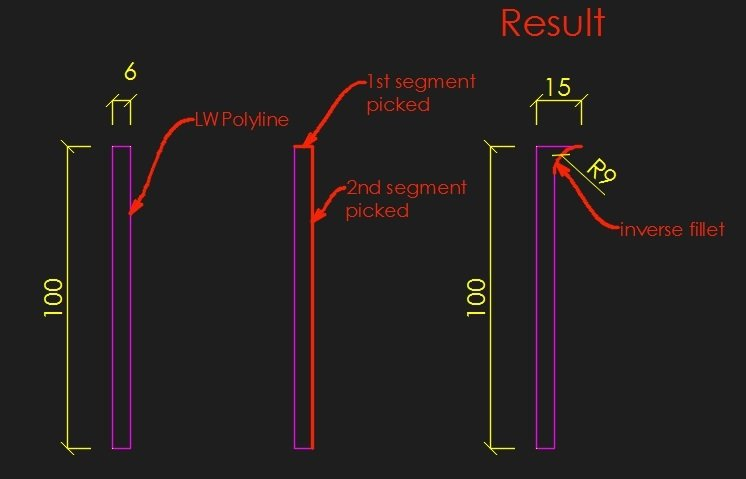##### Share on other sitesMaybe this it is for  two lines you can sort that out I am sure. It was for "Tee" type line intersections that puts an arc on end but not shortening the lines.

Uses multi radio for the flip arc as some day will do an angle check replace with a Y or N if you want.

```; draws an arc between 2 lines but does not erase
; by Alan H Aug 2015
(defun c:arcfill ( / pt1 pt2 pt3 pt4 pt5 obj1 obj2 obj3 obj4 obj5 obj6 rad)
(setq oldsnap (getvar 'osmode))
(setvar 'Osmode 512)
(setq pt1 (getpoint "\npick 1st line for trim near end"))
(setq obj1 (ssname (ssget pt1) 0))
(setq obj5 (vlax-ename->vla-object obj1))
(setq stpt (vlax-curve-getstartpoint obj5 ))
(setq endpt (vlax-curve-getendpoint obj5))
(setq d1 (distance pt1 stpt))
(setq d2 (distance pt1 endpt))

(setq pt2 (getpoint "\npick 2nd line"))
(setq obj2 (ssname (ssget pt2) 0))
(setq obj6 (vlax-ename->vla-object obj2))

(setvar 'osmode 0)
(command "offset" rad Obj1 pt2 "")
(setq obj3 (vlax-ename->vla-object (entlast)))

(command "offset" rad Obj2 pt1 "")
(setq obj4 (vlax-ename->vla-object (entlast)))
(setq pt5 (vlax-invoke obj3 'intersectWith obj4 acExtendThisEntity))
(setq pt3 (vlax-curve-getClosestPointto obj6 pt5))
(setq pt4 (vlax-curve-getClosestPointto obj5 pt5))

(vla-delete obj3)
(vla-delete obj4)
; need a check direction

(command "arc" "C" pt5 pt3 pt4)

(if (= but nil)(setq but 1))
(setq ans (ah:butts but "h"  '("Flip arc" "Yes" "No")))
(if (= ans "Yes")
(progn
(command "erase" "last" "")
(command "arc" "C" pt5 pt4 pt3)
)
)

(if (< d1 d2)
(vla-put-startpoint obj5 (vlax-3d-point pt4))
(vla-put-endpoint obj5 (vlax-3d-point pt4))
)

(setvar 'osmode oldsnap)
)
(c:arcfill)```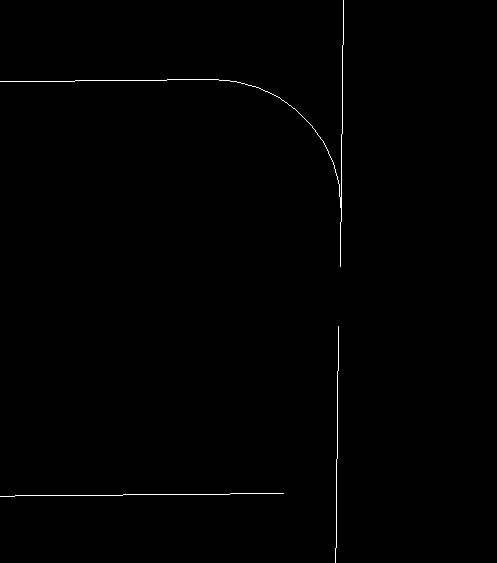##### Share on other sitesPosted (edited)

Gone to get something to eat a start. Getting there.

`Removed see next post`

Got something to eat but realise need a check for clockwise pline at start so for a random pline shape depends on direction drawn.

Needs a bit more debugging need to work on angles a bit more so always goes in correct direction. Problem is in a arc is always anti clockwise.

Edited by BIGAL

##### Share on other sitesPosted (edited)

Hi Grr

Try this I think only works with anti clockwise plines will add that later. Need to add make closed if want to do another corner on same pline.

```; add an arc fillet  to outside of pline rather than inside.
; By Alan H March 2020
; more details info@alanh.com.au

(defun c:plseg( / A90 ANG1 ANG2 ANS CEN CO-ORD NEW_COORD1 OBJ2 OBJ3 OLDSNAP PLENT PT1 PT2 PT3 PT4 PT5  X plent)

(setq a90 (/ pi 2.0))
(setq oldsnap (getvar 'osmode))
(setvar 'osmode 1)
(setq pt1 (getpoint "\nSelect Pline corner for arc "))

(command "circle" pt1 (+ rad 1))
(command "trim" (entlast) "" pt1 "")
(command "erase" (entlast) "")
(setq pt1 (getpoint "\nPick pline end for arc"))

(setq plent (ssname (ssget pt1) 0))
(setq co-ord (mapcar 'cdr (vl-remove-if-not '(lambda (x) (= (car x) 10)) (entget plent))))
(if (equal (list (car pt1)(cadr pt1)) (nth 0 co-ord) 1e-07)
(setq ptis 1)
(setq ptis 2)
)
(setq x (length co-ord))
(setvar 'osmode 0)
(setq ang1 (angle (nth 0 co-ord)(nth 1 co-ord)))
(setq ang2 (angle (nth (- x 1) co-ord)(nth (- x 2) co-ord)))
(if (= ptis 1)
(progn
(setq pt2 (polar (nth  0 co-ord) (- ang1 a90) rad))
(setq pt3 (polar (nth 1 co-ord) (- ang1 a90) rad))
)
(progn
(setq pt2 (polar (nth (- x 1) co-ord) (+ ang2 a90) rad))
(setq pt3 (polar (nth (- x 2) co-ord) (+ ang2 a90) rad))
)
)
(command "line" pt2 pt3 "")
(setq obj2 (entlast))

(if (= ptis 1)
(progn
(setq pt4 (polar (nth (- x 1) co-ord) (- ang2 a90) rad))
(setq pt5 (polar (nth (- x 2) co-ord) (- ang2 a90) rad))
)
(progn
(setq pt4 (polar (nth  0 co-ord) (+ ang1 a90) rad))
(setq pt5 (polar (nth 1 co-ord) (+ ang1 a90) rad))
)
)
(command "line" pt4 pt5 "")
(setq obj3 (entlast))

(setq cen (inters pt2 pt3 pt4 pt5 nil))
(command "erase" obj2 obj3 "")

(if (= ptis 1)
(progn
(command "arc" (nth 0 co-ord)"e" (polar cen (+ ang2  a90)rad) "r" rad)
(command "extend" (entlast) "" (nth  (- x 1) co-ord) "")
)
(progn
(command "arc" (polar cen (- ang1 a90) rad) "e" (nth  (- x 1) co-ord) "r" rad)
(command "extend" (entlast) "" (nth 0  co-ord) "")
)
)
(command "pedit" plent "join" (entlast) "" "")
(setvar 'osmode oldsnap)
(princ)
)
(c:plseg)```

Edited by BIGAL

##### Share on other sitesPosted (edited)
On 3/16/2020 at 4:17 AM, Grrr said:

Hey guys, I'm wondering does anyone have written such routine already -

• Dealing with a LWPOLYLINE

1. Pick 1st segment

2. Pick 2nd segment

4. Extend the 1st or 2nd segment, then apply inverse fillet

Two scenarios CW (clock wise) CCW (counter CW)

which direction?

1.polygon (CW) Pick seg1 & seg2  (CW)

2.polygon (CCW) Pick seg2 & seg1  (CCW)

```(defun c:test (/	rad 2e aa ang da en ep1	ep2 l new p p1 p2 param pl pt r td foo tan )

;reverse fillet
;hanhphuc 09.04.2020

tan '((x) (/ (sin x) (cos x)))
foo '((x / a)
(setq a (abs x))
(if
(>= (- pi a) pi)
(- (* pi 2.) (- pi a))
(- pi a)
)
)
)

(and

(while (or (not r) (zerop r))
(initget 6)
(setq
r	(cond ((getreal
)
)
)
)

)

(while (not 2e)
(setq param	(mapcar	'(lambda (a b)
(mapcar 'set
(list 'en b)
(set a (_entsel "LWPOLYLINE,LINE"))
)
(vlax-curve-getparamatpoint
en
(set b (trans (osnap (eval b) "_nea") 1 0))
)
)
'(ep1 ep2)
'(p1 p2)
)
aa	(mapcar
'(lambda (a b)
(angle '(0. 0. 0.) (vlax-curve-getFirstDeriv (car a) b))
)
(list ep1 ep2)
param
)

2e	(not
(apply
'equal
(append (mapcar '(lambda (x) (abs (cos x))) aa) '(1e-6))
)
)
)

(if	(not 2e)
)

T

)

(progn

(setq pt  (vlax-curve-getpointatparam
en
)
ang (foo (apply '- (reverse aa)))
da  (/ ang 2.)
td  (abs (* r (tan da)))
pl  (mapcar '(lambda (x / p)
(cons 10 (setq p (polar pt x td)))
(mapcar '* '(1.0 1.0) p)
)
aa
)

l   (list
(cons 10 pt)
(cons 10 (car pl))
(cons 42
((if (apply '< aa)
-
+
)
(tan (/ ang 4))
)
)

)

new (entmakex	(vl-list* '(0 . "LWPOLYLINE")
'(100 . "AcDbEntity")
'(100 . "AcDbPolyline")
'(70 . 0)
(cons 90 (length pl))
l
)
)

)

;;;    (vl-cmdf "_TRIM" new "" p2 "")  ;;; trim must be within tangent distance otherwise failed

)

)

(princ)

)

(defun _entsel ( str / sel is )
(setvar 'ERRNO 0)(terpri)
(while (not sel) ;(/= (getvar 'ERRNO) 52)
(if (and (setq sel	(entsel
(strcat "\r"
(cond	((= 7 (getvar 'ERRNO)) "Missed?! ")
(is "Invalid entity! ")
("")
)
"Select "
str
" :          "
)
)
)
;(setq is (/= (cdr (assoc 0 (entget (car sel)))) str))
(setq is (not (wcmatch (cdr (assoc 0 (entget (car sel)))) str)))
)
(progn (setvar 'ERRNO 0) (setq sel nil))
sel
)
)
)```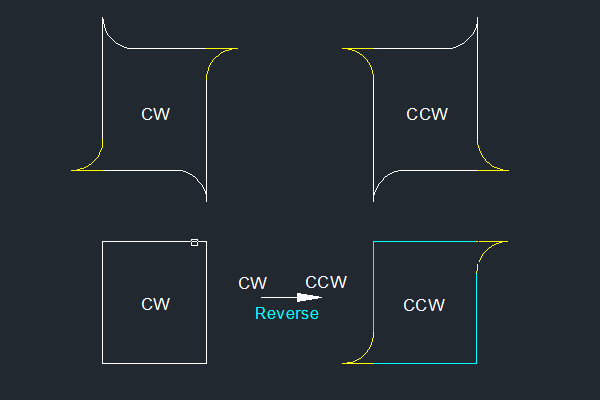Edited by hanhphuc

##### Share on other sitesIt does not clean up the rectang was it meant to ?  Makes fillet on opposite corner ? Started with plain RECTANG.

Where as mine adds arc to pline. Not saying its perfect but seemed to work from the limited testing The CCW and CW is a pain to work around.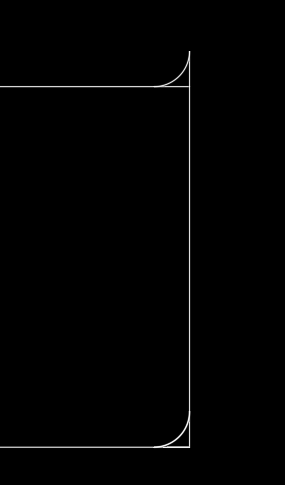•1

##### Share on other sitesPosted (edited)
On 4/10/2020 at 9:39 AM, BIGAL said:

It does not clean up the rectang was it meant to ?  Makes fillet on opposite corner ? Started with plain RECTANG.

Where as mine adds arc to pline. Not saying its perfect but seemed to work from the limited testing The CCW and CW is a pain to work around.

Thanks for pointing out the typos, another was an extra 'x'

```2e	(not
(apply
'equal
(append x (mapcar '(lambda (x) (abs (cos x))) aa) '(1e-6))
)
)```

i've edited :  (append  (mapcar << .... snippet >>

my previous code was just a test which user pick  must follow the direction CW or CCW of the polyline.

agree a bit hiccups need to check if user wanna pick a CW polyline in CCW manner then it needs to be 'reverse',

either use some exsting routine or simply command: REVERSE (AC2012 later?)

Actually if there's only single polyline picked without trim then it is possible (my previous can pick 2 entities)

Here's my workaround filter selection for CW polylines, REVERSE all selected to CCW directions,

then easier all have common directions , modify some codes

lastly restore previous CW selection.

Testing both CCW & CW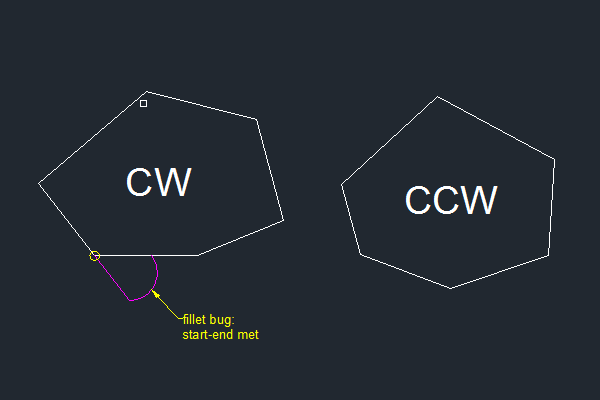some considerations :

1.make sure closed polyline without bulge

2.purge duplicates vertices

3.need to check if fillet tangent larger than segment length

4.common fillet/chamfer restriction, can not fillet at start-end point of polygon

5.support ocs or normal

6.what happen picked segments not adjacent apart?

5.draworder - newly 'filleted' segment put to last?

```(defun c:iftest  ( / *error* 2e  aa ang  cw da en ep1 ep2 foo ls ocs ok p p1 p2 param pl pt r rad rv ss tan td )
;;hanhphuc
;;Invert fillet test
;;v1.1
;;fillet support ocs
;;clockwise & CCW

(defun _entsel ( str / sel is )
(setvar 'ERRNO 0)(terpri)
(while (not sel) ;(/= (getvar 'ERRNO) 52)
(if (and (setq sel	(entsel
(strcat "\r"
(cond	((= 7 (getvar 'ERRNO)) "Missed?! ")
(is "Invalid entity! ")
("")
)
"Select "
str
" :          "
)
)
)
(setq is (/= (cdr (assoc 0 (entget (car sel)))) str))
)
(progn (setvar 'ERRNO 0) (setq sel nil))
sel
)
)
)

tan '((x) (/ (sin x) (cos x))) ; autocad only
foo '((x / a)
(setq a (abs x))
(if
(>= (- pi a) pi)
(- (* pi 2.) (- pi a))
(- pi a)
)
)
rv '((ss)
(mapcar
'revlwpline
(vl-remove-if 'listp (mapcar 'cadr (ssnamex ss)))
)
)
*error* '((msg)
(and ss
(if revlwpline
(vl-cmdf "_REVERSE" ss "")  ;;;autocad only
)
)
(princ " *cancel* ")
)
)

(and
(setq ss (sel-clockwise))
(if revlwpline (rv ss)        ; Bricscad
(vl-cmdf "_REVERSE" ss "")  ;;;autocad only
)
)

(and
(while (or (not r) (zerop r))
(initget 6)
(setq r
(cond
((getreal
)
)
)
)
)

(while (or (not 2e) (not ok))

(if
(vl-some 'not
(setq
param (mapcar
'(lambda (a b)
(mapcar 'set
(list 'en b)
(set a (_entsel "LWPOLYLINE"))
)
(vlax-curve-getparamatpoint
en
(set b (trans (osnap (eval b) "_nea") 1 0))
)
)
'(ep1 ep2)
'(p1 p2)
)
)
)
(setq ok nil)
(setq ocs (cdr (assoc 210 (entget en)))
aa (mapcar
'(lambda (a b)
(angle '(0. 0. 0.)
(trans (vlax-curve-getFirstDeriv (car a) b) 0 ocs)
)
)
(list ep1 ep2)
param
)
2e (not
(apply 'equal
(append
(mapcar '(lambda (x) (abs (cos x))) aa)
'(1e-6)
)
)
)

; if same or polygon
ok (apply 'equal
(mapcar 'car
(list ep1
ep2
)
)
)
)
)

(if (not 2e)
)

T
)

(setq ls
(apply 'append
(mapcar '(lambda (a b)
(setq a (car a))

(list
(vlax-curve-getpointatparam
a
(fix (vlax-curve-getparamatpoint a b))
)

(vlax-curve-getpointatparam
a
(1+ (fix (vlax-curve-getparamatpoint a b)))
)

)
)

(list ep1 ep2)
(list p1 p2)
)
)
)

(progn

pt (vlax-curve-getpointatparam
en
param
(reverse param)
)
)
)
)
pt (trans pt 0 ocs)
ang (foo (apply '-
(reverse (if cw
aa
(mapcar '+ (list pi pi) (reverse aa))
)
)
)
)
da (/ ang 2.)
td (abs (* r (tan da)))
pl (mapcar '(lambda (x / p)
(cons 10 (setq p (polar pt x td)))
(mapcar '* '(1.0 1.0) p)
)
(if cw
aa
(mapcar '+ (list pi pi) aa)
)
)
)

(entmakex
(list
'(0 . "LWPOLYLINE")
'(100 . "AcDbEntity")
'(100 . "AcDbPolyline")
'(70 . 0)
(assoc 38 (entget en))
(cons 90 (length pl))
(cons 10 pt)
(cons 10 (car pl))
(cons 42
((if (or cw (apply '< aa))
-
+
)
(tan (/ ang 4))
)
)
(cons 210 ocs)
)
)
)

(and
ss
(if revlwpline (rv ss)  ; Bricscad
(vl-cmdf "_REVERSE" ss "") ;;;autocad only
)
;; (sssetfirst nil ss)
)

)

(princ)

)

;; List Clockwise-p - Lee Mac
;; Returns T if the point list is clockwise oriented
(defun LM:ListClockwise-p ( lst )
(minusp
(apply '+
(mapcar
(function
(lambda ( a b )
)
)
lst (cons (last lst) lst)
)
)
)
)

(defun sel-clockwise (/ ss)

(and
(setq ss  (ssget "_X" '((0 . "LWPOLYLINE")(410 . "Model"))))
(vl-remove nil
(mapcar
'(lambda (en)
(if (not (LM:listclockwise-p
(mapcar
'cdr
(vl-remove-if
'(lambda (x) (/= (car x) 10))
(entget en)
)
)
)
)
(progn
(ssdel en ss) nil
)
en
)
)
(vl-remove-if 'listp (mapcar 'cadr (ssnamex ss)))
)
)

;(sssetfirst nil ss)

)
ss
)```

p/s: i have a better way easier than this without checking CCW CW, supports bulge & end-point bugs.

Edited by hanhphuc
v1.1 code updated

##### Share on other sitesPosted (edited)

Its a hard task thats why did pick which leg, Bulges not a problem in mine provide the pline is set to closed else trim cuts pline in to 2 so crashes. Obviously can not fillet a bulge.

I found a mistake as well, code updated.

(vla-put-closed (vlax-ename->vla-object (entlast)) -1)

Neded after pedit to make sure it is closed if do another corner.

Edited by BIGAL

##### Share on other sitesOn 4/12/2020 at 7:42 AM, BIGAL said:

Its a hard task thats why did pick which leg, Bulges not a problem in mine provide the pline is set to closed else trim cuts pline in to 2 so crashes. Obviously can not fillet a bulge.

hi @BIGAL Have you tried trim command vs non planar condition (2 lines L1 & L2)?

Example: Radius=5, fillet L1 & L2

The difference, yellow dotted normal UCS (0 0 1) where L1 & L2, z=elevations are all same

see the 'filleted' cyan Arc is planar to both L1 & L2  (i.e: end points different z elevations)

i check Bricscad fillet command solves non-planar,

whereas AutoCAD 2007 fillet L1 L2 skips due to non-planar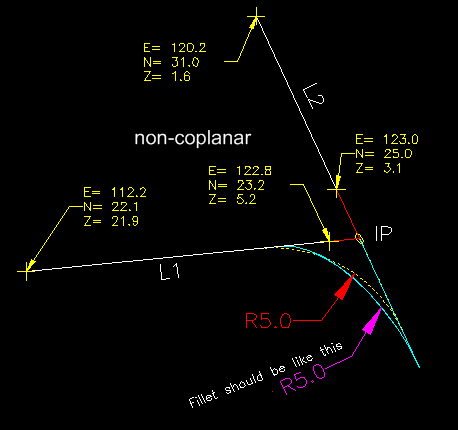my \$0.02 solution is with bulge conversion,

further topic discussions --> Here

p/s: isn't "Extend Fillet" is more appropriate?

my \$0.02 inverse definition is either 'trimmed' fillet inside or 'mirrored' out side##### Share on other sitesAs you say once you introduce a 3rd dimension it all changes what is the correct arc plane ?

If you look at a staircase handrail a good example of drawing in correct plane as it turns say 90 .

My version will probably not work, but it was based around Grrr original request.

Hey Grrr did you try both codes, which one did you like  or did it fail ?

##### Share on other sitesHey boys,

Kinda late in the topic, but seems like a fun one to crack. This one is done by pure mathematics calculation:

```;; Inverse Fillet --> Jonathan Handojo
;; Performs a fillet on the opposite side as opposed to the FILLET command.
;; Select two lines of the same polyline, and specify radius.

(defun c:ifillet ( / *error* acadobj activeundo adoc ang3pt arcang bulgeang clen clock-p coff ent1 ent1closest ent2 ent2closest i msp n newpt np1 np3 offslen p1 p2 p3 pl pts rad seg1 seg2 segs unq vent1)
(defun clen (ang r) (* 2 r (sin (* 0.5 ang))))
(defun coff (ang r) (/ (clen ang r) (sqrt (- 2 (* 2 (cos (- pi ang)))))))
(defun bulgeang (ang) (/ (sin (/ ang 4)) (cos (/ ang 4))))
(defun arcang (chordlength r) (acos (/ (- (* 2 (expt r 2)) (expt chordlength 2)) (* 2 (expt r 2)))))
(defun ang3pt (p1 p2 p3)
((lambda (x) (if (> x pi) (- (* 2 pi) x) x)) (abs (- (angle p2 p3) (angle p2 p1))))
)

(defun *error* ( msg )
(if (not (wcmatch (strcase msg T) "*break*,*cancel*,*exit*"))
(princ (strcat "Error: " msg))
)
)
activeundo nil)
(if (= 0 (logand 8 (getvar "UNDOCTL"))) (vla-StartUndoMark adoc) (setq activeundo T))

(mapcar '(lambda (x y)
(while
(progn
(set x (entsel y))
(cond
((null (eval x)) (princ "\nNothing selected"))
((not (wcmatch (cdr (assoc 0 (entget (car (eval x))))) "LINE,LWPOLYLINE")) (princ "\nObject is not a line or polyline"))
)
)
)
)
'(ent1 ent2)
'("\nSelect first line: " "\nSelect second line: ")
)

(setq ent1closest (vlax-curve-getclosestpointto (car ent1) (last ent1))
ent2closest (vlax-curve-getclosestpointto (car ent2) (last ent2))
)
(cond
((eq (car ent1) (car ent2))
(setq pts (mapcar 'cdr (vl-remove-if-not '(lambda (x) (eq (car x) 10)) (entget (car ent1))))
pl (JH:RemoveAdjacentDuplicates (append pts (list (car pts))))
segs (mapcar '(lambda (x) (cons (car pl) (car (setq pl (cdr pl))))) (cdr pl))
)
(mapcar '(lambda (x y z)
(set x (car
(vl-member-if
'(lambda (a)
(<= (if (equal a (car segs)) (vlax-curve-getStartParam (car y)) (vlax-curve-getParamAtPoint (car y) (car a)))
(vlax-curve-getParamAtPoint (car y) z)
(if (equal a (last segs)) (vlax-curve-getEndParam (car y)) (vlax-curve-getParamAtPoint (car y) (cdr a)))
)
)
segs
)
)
)
)
'(seg1 seg2)
(list ent1 ent2)
(list ent1closest ent2closest)
)
(setq p2 (vl-some '(lambda (x) (if (null (= (- (length unq) (length (vl-remove-if '(lambda (y) (equal x y 1e-8)) unq))) 1)) x)) (setq unq (list (car seg1) (cdr seg1) (car seg2) (cdr seg2))))
p1 (car (vl-remove-if '(lambda (x) (equal x p2 1e-8)) (list (car seg1) (cdr seg1))))
p3 (car (vl-remove-if '(lambda (x) (equal x p2 1e-8)) (list (car seg2) (cdr seg2))))
offslen (coff (ang3pt p1 p2 p3) rad)
np1 (polar p2 (angle p1 p2) offslen)
np3 (polar p2 (angle p2 p3) offslen)
clock-p (equal (angle p1 p2)
(angle '(0 0 0)
(vlax-curve-getFirstDeriv (car ent1)
(vlax-curve-getParamAtPoint (car ent1) ent1closest)
)
)
1e-8
)
newpt (subst
np1
(if clock-p
(car seg2)
(car seg1)
)
(mapcar 'car segs)
)
newpt ((lambda (a n l / i lst)	; <--- Little did I know that it's exactly the same as LM:insertnth when I wrote without looking at it. Too late by the time I realised
(setq i -1)
(if (equal l (setq lst (apply 'append (mapcar '(lambda (x) (if (= (setq i (1+ i)) n) (list a x) (list x))) l))))
(append l (list a))	; <--- Tweaked a bit though
lst
)
)
np3
((if clock-p 1+ +)
(vl-position np1 newpt)
)
newpt
)
)
(vlax-put (setq vent1 (vlax-ename->vla-object (car ent1))) 'Coordinates (apply 'append newpt))
(vla-SetBulge vent1 ((if clock-p + 1-)
(vl-position np1 newpt)
)
((if (apply 'LM:Clockwise-p
(vl-sort (list p1 p2 p3)
'(lambda (a b)
(< (vlax-curve-getParamAtPoint (car ent1) a)
(vlax-curve-getParamAtPoint (car ent1) b)
)
)
)
)
+ -) (bulgeang (arcang (distance np1 np3) rad)))
)

)
)

(princ)
)

;; Removes points that gives zero-lengths between them
;; Example call: JH:RemoveAdjacentDuplicates (a b c c d e e f a a g) ---> (a b c d e f a g)
;; Doing ssget F, WP, CP, or other requiring list of point will return nil if adjacent duplicates are found
;; lst - list of points

(vl-remove-if '(lambda (x) (equal x (car (setq lst (cdr lst))) 1e-8)) lst)
)

;; ArcCosine  -  Lee Mac
;; Args: -1 <= x <= 1

(defun acos ( x )
(if (<= -1.0 x 1.0)
(atan (sqrt (- 1.0 (* x x))) x)
)
)

;; Clockwise-p - Lee Mac
;; Returns T if p1,p2,p3 are clockwise oriented

(defun LM:Clockwise-p ( p1 p2 p3 )
(<
)
)```

Even breaking down the (command "FILLET") command is pretty extreme, and this is just polyline, not considering other curves...

##### Share on other sites6 hours ago, BIGAL said:

As you say once you introduce a 3rd dimension it all changes what is the correct arc plane ?

If you look at a staircase handrail a good example of drawing in correct plane as it turns say 90 .

My version will probably not work, but it was based around Grrr original request.

Hey Grrr did you try both codes, which one did you like  or did it fail ?

no worries Alan, as long as we are healthy, many ways to skin the catstay safe always, lockdown gets over soonsample DWG

## Join the conversation

You can post now and register later. If you have an account, sign in now to post with your account.
Note: Your post will require moderator approval before it will be visible.×   Pasted as rich text.   Paste as plain text instead

Only 75 emoji are allowed.